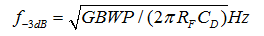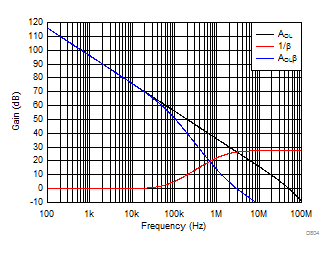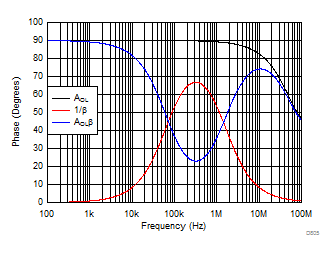JAJSFO4B August   2017  – December 2018

PRODUCTION DATA.

1. 特長
2. アプリケーション
3. 概要
4. 改訂履歴
5. Pin Configuration and Functions
6. Specifications
7. Detailed Description
1. 7.1 Overview
2. 7.2 Functional Block Diagram
3. 7.3 Feature Description
4. 7.4 Device Functional Modes
8. Application and Implementation
1. 8.1 Application Information
2. 8.2 Typical Applications
1. 8.2.1 Transimpedance Amplifier
2. 8.2.2 Multichannel Sensor Interface
9. Power Supply Recommendations
10. 10Layout
1. 10.1 Layout Guidelines
2. 10.2 Layout Example
11. 11デバイスおよびドキュメントのサポート
12. 12メカニカル、パッケージ、および注文情報

• D|8
• DCN|8
• DGK|8
• DCN|8
• DGK|8

#### 8.2.1.2 Detailed Design Procedure

Designs that require high bandwidth from a large area detector with relatively high transimpedance gain benefit from the low input voltage noise of the OPA2810. This input voltage noise is peaked up over frequency by the diode source capacitance, and can, in many cases, become the limiting factor to input sensitivity. The key elements to the design are the expected diode capacitance (CD) with the reverse bias voltage (VBIAS) applied, the desired transimpedance gain, RF, and the GBWP for the OPA2810 (70 MHz). Figure 74 shows a transimpedance circuit with the parameters as described in Table 3. With these three variables set (and including the parasitic input capacitance for the OPA2810 and the PCB added to CD), the feedback capacitor value (CF) may be set to control the frequency response. Transimpedance Considerations for High-Speed Amplifiers application report discusses using high-speed amplifiers for transimpedance applications. To achieve a maximally-flat second-order Butterworth frequency response, set the feedback pole to:

Equation 7.The input capacitance of the amplifier is the sum of the common-mode and differential capacitance (2.5 + 0.5) pF. The parasitic capacitance from the photodiode package and the PCB is approximately 0.3 pF. Using Equation 3, this results in a total input capacitance of CD = 23.3 pF. From Equation 7, set the feedback pole at 1.55 MHz. Setting the pole at 1.55 MHz requires a total feedback capacitance of 1.03 pF.

The approximate –3-dB bandwidth of the transimpedance amplifier circuit is shown in:

Equation 8.Equation 8 estimates a closed-loop bandwidth of 2.19 MHz. Figure 75 and Figure 76 show the loop-gain magnitude and phase plots from the TINA-TI simulations of the transimpedance amplifier circuit of Figure 74. The 1/β gain curve has a zero from RF and CIN at 70 kHz and a pole from RF and CF cancelling the 1/β zero at 1.5 MHz resulting in a 20 dB/decade rate-of-closure at the loop gain crossover frequency (freqeuncy where AOL = 1/β), ensuring a stable circuit. A phase margin of 62° is obtained with a closed-loop bandwidth of 3 MHz and a 100-kΩ transimpedance gain.Figure 75. Loop-Gain Magnitude vs Frequency for Transimpedance Amplifier Circuit of Figure 74Figure 76. Loop-Gain Phase vs Frequency for Transimpedance Amplifier Circuit of Figure 74